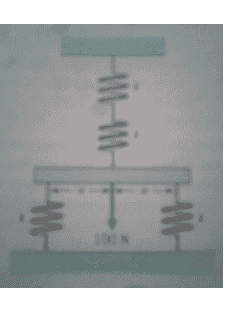# Need some help with this spring system question.

Infernorage

## Homework Statement

A very stiff horizontal bar, supported by four identical springs, as shown in the figure below, is subjected to a center load of 100N. What load is applied to each spring?Repeat the previous problem, except assume that the horizontal bar as configured is not rigid and also has a spring constant of k.

2. The attempt at a solution
So I figured out the first question and the resulting equations were F1(force in the lower springs)=kx1=2/5*F and F2(force in the upper springs)=1/2kx1=1/5F. Just subbing in 100N for F, the force in the lower springs came out to be 40N and 20N in the upper springs.

My problem is with the second question. I am not really sure how to do the problem when the bar has a spring constant. I thought about just using the same equations I came to above, but instead of F being 100N it would have to be some equation involving the spring constant k and deflection, but I am not sure if that is the correct direction. If it is the correct direction, then what would the equation be for the force in that bar?

Thanks in advance for the help.

Infernorage
Can anyone help me out with this? The assignment with this question is due tomorrow morning so I need help quick.